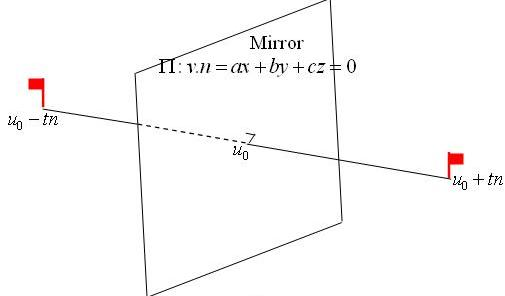#### You may also likeA quadrilateral changes shape with the edge lengths constant. Show the scalar product of the diagonals is constant. If the diagonals are perpendicular in one position are they always perpendicular?As a quadrilateral Q is deformed (keeping the edge lengths constnt) the diagonals and the angle X between them change. Prove that the area of Q is proportional to tanX.### Air Routes

Find the distance of the shortest air route at an altitude of 6000 metres between London and Cape Town given the latitudes and longitudes. A simple application of scalar products of vectors.

# Quaternions and Reflections

##### Age 16 to 18 Challenge Level:

Note that the quaternion product explored here (of two 4-dimensional numbers) is simply a combination of the scalar product and the vector product of the corresponding vectors in 3-dimensional space which explains where the definitions of these products of vectors comes from.You need to know that, as $v = u_0$ is a point on the mirror-plane $\Pi$, by simply substituting the co-ordinates of the point in the equation of the plane, you get $u_0\cdot n =0$.

The first two parts have been solved by Andrei of Tudor Vianu National College, Bucharest, Romania.

(1)We first multiply the pure quaternions: $v_1 = x_1i + y_1j + z_1k$ and $v_2 = x_2i + y_2j + z_2k.$ to obtain: $$v_1v_2 = -x_1x_2 - y_1y_2 - z_1z_2 + (y_1z_2 - y_2z_1)i + (z_1x_2 - x_1z_2)j + (x_1y_2 - y_1x_2)k .$$ The scalar product is: $v_1\cdot v_2 = x_1x_2 + y_1y_2 + z_1z_2$ and the vector product is: $$v_1 \times v_2 = (y_1z_2 - y_2z_1)i + (z_1x_2 + x_1z_2)j + (x_1y_2 - y_1x_2)k$$ We observe that the quaternion product is a combination of the scalar product and the vector product of the corresponding vectors in $R^3$, that is: $v_1v_2 = -( v_1 \cdot v_2) + (v_1 \times v_2)$

(2) Now, considering all the points on the unit sphere $v = xi + yj + zk$ where $|v| = \sqrt (x^2 + y^2 + z^2) = 1$, we calculate $v^2$. We find $v^2 = -x^2 -y^2 - z^2 = -1$ so there are infinitely square roots of -1 in $R^3$.

In an alternative notation the points on the unit sphere are given by: $v = \cos \theta \cos \phi i + \cos \theta \sin \phi j + \sin^2 \theta k$ where $|v| = \sqrt (\cos^2 \theta \cos^2 \phi + \cos^2 \theta \sin^2 \phi + \sin^2\theta) = 1$.

The solution to the third part is as follows:

(3) $u_0n = -u_0\cdot n + u_0\times n = -0 + u_0\times n = 0 + -n\times u_0 = u_0\cdot n -n\times u_0 = -nu_0$ therefore $F(u_0) = nu_0n = n(-nu_0) = -n^2u_0 = u_0$

$F(u_0 + tn) = n(u_0 + tn)n = nu_0n + ntn^2 = u_0 + tn^3 = u_0 - tn$. Any point in $\mathbb{R}^3$ can be written as a sum $u_0 + tn$ for some $u_0$ in $\Pi$ and some $t\geq 0$, so $F$ gives a reflection in $\Pi$.